# SAT Math Multiple Choice Question 676: Answer and Explanation

### Test Information

Question: 676

1. A jar contains only red, white, and blue marbles. It contains twice as many red marbles as white marbles and three times as many white marbles as blue marbles. If a marble is chosen at random, what is the probability that it is not red?

• A.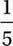• B.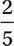• C.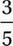• D.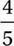Let R = the number of red marbles, W = the number of white marbles, and B = the number of blue marbles. If the jar contains twice as many red marbles as white marbles, then R = 2W. If the jar contains three times as many white marbles as blue marbles, then W = 3B. We can substitute numbers to these equations to solve the problem. Let's say B = 10. This means there are 3(10) = 30 white marbles and 2(30) = 60 red marbles. The total number of marbles is therefore 10 + 30 + 60 = 100, and the number of non-red marbles is therefore 10 + 30 = 40 marbles, so the probability that the marble is not red is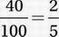.